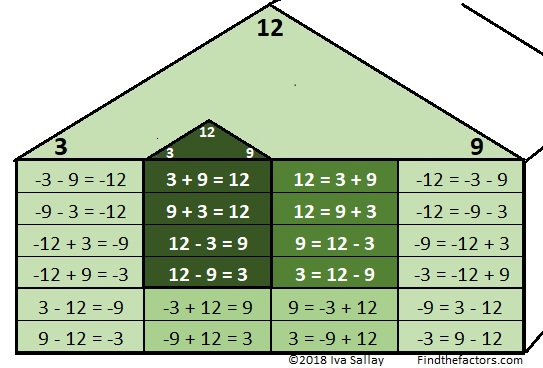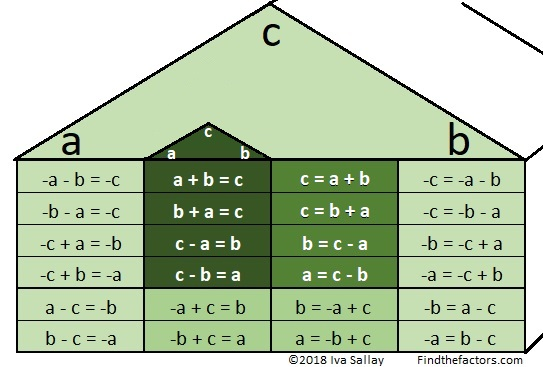# 1239 Addition and Subtraction Families

Perhaps in the early years of your education, you were introduced to addition and subtraction families. For example, you might have made a little house out of these four addition and subtraction facts:

3 + 9 = 12
9 + 3 = 12
12 – 9 = 3
12 – 3 = 9

You should have been told then, but weren’t, that there are other members of this addition and subtraction family:

12 = 3 + 9
12 = 9 + 3
3 = 12 – 9
9 = 12 – 3

In fact, those second four addition and subtraction facts may have seemed very strange-looking even years later.

Eventually, you should have been introduced to the whole family of facts involving addition and subtraction and those numbers, but most likely that never happened. Here, the most familiar part of the family can be seen in the dark green part of the house, but the entire rest of the family can also be seen throughout the rest of the house. Some are in the basement and some in the wings of the house, but they all very much belong in this addition and subtraction family home. And it isn’t too difficult to see where every member of the family came from:If you knew all the members of that family, it would be more natural to accept the members of a family made with variables or numbers mixed with variables:Instead, many students get very confused when they become teenagers and are introduced to families in which some family members are numbers and some are letters. Likewise, some family members are positive and some are negative.

Going from “a + b = c” to “c – a = b” becomes confusing instead of natural. Required steps involve adding and subtracting the same value from both sides of the equation instead of recalling prior knowledge known since first grade.

I think middle school students might benefit from building an addition and subtraction family house.

Now I would like to share some facts about the number 1239:

• 1239 is a composite number.
• Prime factorization: 1239 = 3 × 7 × 59
• The exponents in the prime factorization are 1, 1, and 1. Adding one to each and multiplying we get (1 + 1)(1 + 1)(1 + 1) = 2 × 2 × 2 = 8. Therefore 1239 has exactly 8 factors.
• Factors of 1239: 1, 3, 7, 21, 59, 177, 413, 1239
• Factor pairs: 1239 = 1 × 1239, 3 × 413, 7 × 177, or 21 × 59
• 1239 has no square factors that allow its square root to be simplified. √1239 ≈ 35.199431239 is divisible by 3 because it is made with three consecutive numbers and a multiple of 3. In this case it isn’t necessary to add the numbers up to see that.

This site uses Akismet to reduce spam. Learn how your comment data is processed.